• Latex 表格中插入公式出现Missing { inserted. &$C\xrightarrow{(n^',d_1^',p^')} 千次阅读 2019-12-10 08:58:39 写了个表格，在插入的公式的地方（第9行）总是报一堆类似的 Missing { inserted. &$C\xrightarrow{(n^',d_1^',p^')}

错误。查了好久也没解决。在今天早晨灵光乍现，突然就改好了。以下是错误代码。

\begin{table}[]\label{comm}
\caption{Communication cost of client and cloud/edge server in SoSQC1 and SoSQC2}
\begin{tabular}{@{}lllll@{}}

\toprule
$Algorithm$ & $Transmission\ data$ & $Data\ size$ & \multicolumn{2}{l}{$Total$}  \\
\midrule
\multirow{5}{*}{$SoSQC1$}
&$C\xrightarrow{(n^',d_1^',p^')}S$& \multicolumn{1}{l}{$6\ (Len)$} & \multirow{2}{*}{$C\to S:$} & \multirow{2}{*}{$9+2Num\ (Len)$}  \\

\multicolumn{1}{c}{}& $C\xrightarrow{(a^{'})}S$ & $2Num\ (Len)$ & \multirow{3}*{$S\to C:$} & \multirow{3}*{$2+2Num\ (Len)$}  \\
\multicolumn{1}{c}{}& $S \xrightarrow{(R_1^{'})}C$ & $2Num\ (Len)$ & & \\
\multicolumn{1}{c}{}& $C \xrightarrow{(\omega,d_2^{'})}S$ &$3\ (Len)$& & \\
\multicolumn{1}{c}{}& $S \xrightarrow{(R_2^{'})}C$ &$2\ (Len)$&  &\\
\midrule
\multirow{4}{*}{$SoSQC2$}
& $C \xrightarrow{(n^{'},d^{'},d_2^{'},p^{'})}S$ & $7\ (Len)$ & \multirow{1}{*}{$C\to S:$} &\multirow{1}{*}{$7\ (Len)$} \\
& $S \xrightarrow{(R_1^{'})}C$ & $2Num\ (Len)$ & \multirow{2}{*}{$S\to C:$} & \multirow{2}{*}{$3+2Num\ (Len)$} \\

& $S \xrightarrow{(a,R_2^{'})}C$ & $3\ (Len)$ &  &\\
\bottomrule

\end{tabular}
\end{table}

原因是你在表格中插入带有上标之类的符号不能简写。例如，平时$n^'$是没问题的，但是在表格中就会报错。修改方法就是加{}。改成： $n^{'}$ .

所以我在思考，遇到Missing { inserted.  的问题，应该好好考虑哪里缺了或者需要加 {

更多相关内容
• 今天帮别人写Latex格式的报告，发现之前踩过的坑又踩了一遍，特此总结一下。 插公式 强烈安利Mathpix Snipping Tool，这是一款基于OCR文字识别的自动生成公式软件，节约了很多盲目打公式的时间。结合Typora也能生成...

今天帮别人写Latex格式的报告，发现之前踩过的坑又踩了一遍，特此总结一下。

# 插公式

强烈安利Mathpix Snipping Tool，这是一款基于OCR文字识别的自动生成公式软件，节约了很多盲目打公式的时间。结合Typora也能生成Mathtype/ Word公式等其他格式。

打公式怎么自定义编号？

	\begin{equation}
K=\alpha F_{0} \tag{111}
\end{equation}


加\tag{}即可，下面是效果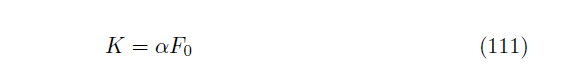# 插图片

怎么一行插入多张图片？

图片和文字的顺序不对？

% \usepackage{graphicx}
% \usepackage{subfigure}
\begin{figure}[H]
\centering
\subfigure{
%\label{fig:subfig:a} %% label for first subfigure
\includegraphics[width=2.3in]{Figures/11.jpg}}
\subfigure{
%\label{fig:subfig:b} %% label for second subfigure
\includegraphics[width=2.3in]{Figures/12.jpg}}
\end{figure}


这是一行两张图的效果，加\会自动换行. width如果太大也会自动换行.
\begin{figure}[H] 中的 [H] 表示它不会强制给你改变文字和图片的顺序.

# 插表格

插入表格也有神器，表格生成器TablesGenerator. 只需要把Excel中的表格选中，复制粘贴到生成器的表格页面，点Generate就可以生成表格对应的Latex代码了。
但是生成的表格会丢失一些竖线，有时候又需要100%还原.
表格中的横线和竖线在哪里加？

	\begin{center}
\begin{tabular}{|l|l|l|l|}
\hline
\textit{$\alpha$} & Strike Price K & \textit{$V_{D}(0)$} & Rider Fees \\ \hline
(6 dps)    & (\% to 4 dps)  &                 &            \\ \hline
0.8        & 80000          & 1.65E+06        & 3.71\%     \\ \hline
0.9        & 90000          & 1.29E+06        & 1.64\%     \\ \hline
1          & 100000         & 9.33E+05        & 0.53\%     \\ \hline
1.1        & 110000         & 5.75E+05        & 0.31\%     \\ \hline
\end{tabular}
\end{center}


效果：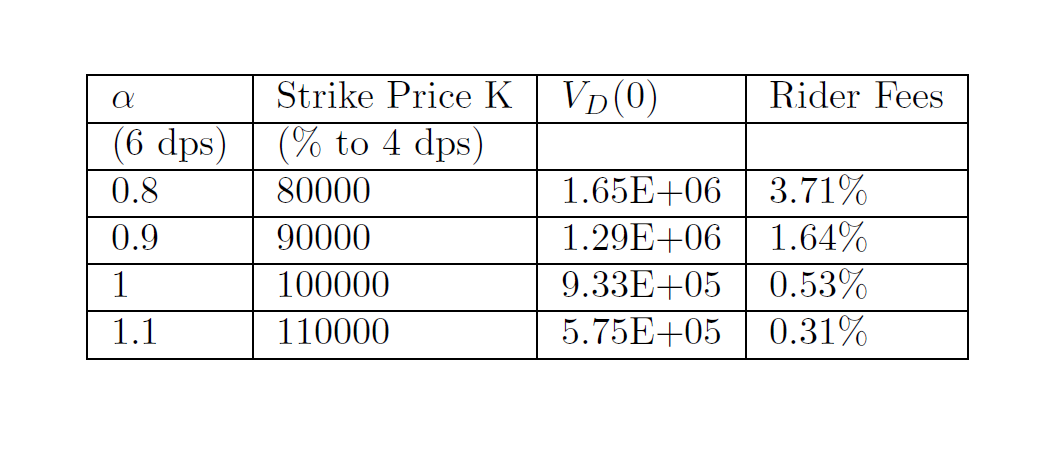\begin{tabular}{|l|l|l|l|}中，字母l代表数据都是左对齐的，字母之间的分割线|就是列的竖线，如果去掉就没有竖线了.
每一行的最后写一个\ \hline，代表换行和加横线.

# 小细节

1. &，% 等符号，使用/&, /%显示
2. 换行\\，换页\newpage
3. 有时候公式、表格什么的连在一起，中间没有文字，觉得公式表格或者图片之间离得太近了。这是不能直接用\\，它会报错说没有文字. 这时使用~\\即可。
展开全文• 美赛LaTeX论文编程关键操作--表格绘制，图片、公式插入

后天2022年美赛就开始了，针对写作要求，博主着重整理了一下运用LaTeX绘制表格，插入图片、公式的详细操作以及相应的注释要求。（以下代码直接复制便可以使用，编号格式方面以及设置好了）

希望大家都能在2022的美赛取得个好成绩哦！

目录

1.绘制表格

1.1代码块如下：

1.2例图：

1.3详解：

2.插入图片

2.1代码如下：

2.2例图：

2.3详解：

3.插入公式

3.1代码如下：

3.2例图：

3.3详解：

## 1.绘制表格

### 1.1代码块如下：

\begin{table}[htbp]
\centering
\caption{Symbols and Definition}
\label{tab:my_label}
\begin{tabular}{c|c}
Symbols&Definition\\
\hline
PEI&The extent to which plastic processing affects the environment\\
PODI&The degree to which plastic processing breaks the environment\\
POEI&How much plastic handling optimises the environment\\
PPRO&Plastic production\\
PP&Plastic pollution levels
\end{tabular}
\end{table}

### 1.2例图：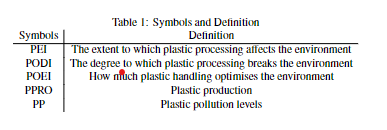### 1.3详解：

表格的插入不需要额外的宏包

\caption{Symbols and Definition}大括号内可以设置表格标题

边框的设置在LaTeX中很有趣，他的纵向线条是运用格式预设的，而横向线条是运用语句\hline绘制的。c|c指的便是两列，而|c|c|指的也是两列，不过后者有外边框，这一点应该很容易理解。而我在输入第一行Symbols……后输入了语句\hline，则在Symbols……下方绘制了一条纵向。纵线的绘制是任意的，在任何一条语句（包括Symbols……）的上下方都可以绘制横线，并且会自动与内容的多少匹配。

## 2.插入图片

### 2.1代码如下：

\begin{figure}[htbp]
\centering
\includegraphics{Model 1.png}
\caption{Model 1}
\label{fig:my_label}
\end{figure}

### 2.2例图：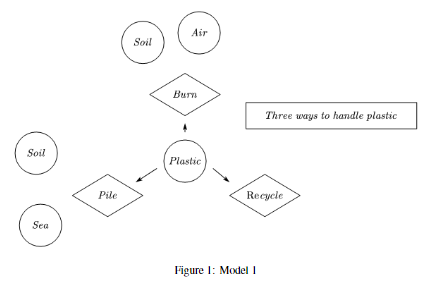### 2.3详解：

首先需要添加宏包\usepackage{graphicx}

\caption{Model 1}大括号内可以设置标题

\includegraphics{Model 1.png}大括号内放置图片的名称。博主用的是网页版的TeX编辑器，文件名称很好查。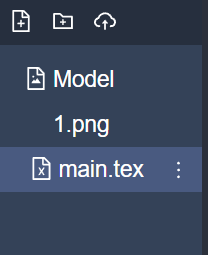## 3.插入公式

### 3.1代码如下：

\begin{equation}
AP=\sum_{n}{\gamma_n\omega_mEM}
\end{equation}

### 3.2例图：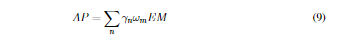### 3.3详解：

首先也是添加宏包\usepackage{amsmath, amsfonts}

格式问题equation以及解决好了，关键便是数学公式的书写，这里推荐一位博主的博文，写的非常详细：LaTeX数学公式-详细教程_NSJim的博客-CSDN博客_latex数学公式

展开全文• 包： \usepackage{booktabs} % 设置加粗的表格线 \usepackage{threeparttable} % ...\usepackage{multirow} % 合并表格中的单元格 \usepackage{diagbox} % 添加斜线表头 \usepackage{array} % 需要该宏包 设置表单元...

用到的包：

\usepackage{booktabs}             % 设置加粗的表格线
\usepackage{threeparttable}       % 给表格添加注释
\usepackage{multirow}     % 合并表格中的单元格
\usepackage{diagbox}    % 添加斜线表头
\usepackage{array}      % 需要该宏包 设置表单元格的高度
\usepackage{caption}    % 设置表格标题居中，单元格内容居左等
\usepackage{colortbl}
\usepackage{longtable}   % 跨页（长）表格

\usepackage{amsmath,amssymb}   % 数学常用环境的汉化
\usepackage{mathrsfs}
\usepackage{IEEEtrantools}


===== 分割线 =============

代码：

\begin{center}
\renewcommand\arraystretch{2}    % 设置高度
\begin{longtable}{|c|c|l|r|l|l|}
\caption{test} \label{tab:5.1} \\
\hline \hline
A & B & \multicolumn{1}{c|}{C} & \multicolumn{1}{c|}{D} & \multicolumn{1}{c|}{E} & \multicolumn{1}{c|}{F}  \\ \hline \hline

\caption[]{(continued)}  \\
\hline \hline
A & B & \multicolumn{1}{c|}{C} & \multicolumn{1}{c|}{D} & \multicolumn{1}{c|}{E} & \multicolumn{1}{c|}{F}  \\ \hline \hline
\hline
% This & goes& at& the& bottom.& & \\
\hline
\endfoot
\hline
% These lines&  will appear& in place& of the usual& foot at the& end of& the table \\
\hline
\endlastfoot

\multirow{4}{*}{ \begin{IEEEeqnarraybox}{s.t}      % {居左，居中}
$a = a+e$ & $d = e$ \\
$b = c$ & $s = f$
\end{IEEEeqnarraybox}
} & 1 & $\gamma$ & $\alpha$ & ABC &   \\ \cline{2-6}
& 2 & $\alpha$ & $\alpha$ & ER &   \\ \cline{2-6}
& 3 & $\alpha$ & $\alpha$ & ER &   \\ \cline{2-6}
& 4 & $\alpha$ & $\alpha$ & WE &
$\begin{pmatrix} 1.2 &1.6096 &0 \\ 1.6096 & 2.3651 &0 \\ 0 & 0 & 1.6805 \end{pmatrix}$  \\ \cline{2-6}
\end{longtable}
\end{center}



% 不能直接在表格中插入文本中的那种多行公式。

% 说明：表格中嵌入多行公式：原则是表格中再放入表格，如上面 \begin{IEEEeqnarraybox} 一种表格；\begin{matrix} % matrix系列 也是一种表格，它们可以直接放在表格中。

效果：展开全文• 在单元格内，需要加上括号，如下： &$$\alpha = 2.0$$ \\
• 写机器学习相关的博客时，插入一些复杂的数学公式是不可避免的事，总是截图并不是一个完美的解决方法，在此总结一下如何使用LaTeX编辑数学公式，包括常用的符号，以及机器学习经常用到的公式。 什么是LaTeX LaTeX...
• 1.插入公式。 2.添加引用。 2.1.使用bib文件添加。 2.2.直接在文章结尾处手动添加 3.运行时提示错误的一些解决方法。 4.其他操作 1.插入公式 公式分为行内公式与单行公式。 LaTex插入的行内公式用$来表示...经验分享 • 请问为什么会出现错误，我实在不知道错在哪里。 以下是凑字数64949767919494649 • LaTex 插入数学公式 共有两种方式，分别是行内公式和行间公式 示例代码如下： 行内公式直接加入$符号 $G=(V,E,A)$是加权有向图（或无向图） 行间公式如下所示： \begin{equation} N_J:=U_{i\in J}N_i=\{j\in L:i\...
• 1、插入表格使用 \begin{tabular}{l c c c r} content... \end{tabular} 其中l表示左对齐，c表示居中对齐，r表示右对齐 2、添加元素，用&符号分割各个名称 \begin{tabular}{l c c c c r} method & ...python
• 需求 Origin本身可以插入简单的公式，但是... 教程 官方教程：Introducing the LaTeX App 安装教程：在Origin绘图和表格中插入Latex公式 详细的使用教程：Origin如何使用LaTex绘制带有高质量公式的图形 效果示意图
• 关于Origin与Latex结合应用 下载: origin latex app 下载 使用教程 origin安装app教程 官方教程 参考 https://baijiahao.baidu.com/s?id=1666395737690093701&wfr=spider&for=pc
• 文章目录公式编辑器表格编辑器Latex设置表格字体大小LateX表格加标题和标签LateX插入三线表 如果在LateX中直接输入、编辑公式表格，不是不可以，但是不能直接可视化编辑，所以会比较痛苦。 以下是我常用、收藏...TeXstudio
• 图表的制作是许多LaTeX用户比较烦恼的问题，但同时也是最迷人的部分，教程将会分几部分进行。看了这篇文章你可以Get到的知识点： tabular环境和array环境...一般使用tabular环境排版表格；array环境：array环境的..ieee论文
• latex-英文排版工具，国外期刊都要求latex排版进行投稿，就像国内要求word排版一样，但是需要公式编辑的时候，是不是又烦躁又觉得麻烦而且问题是不熟悉latex公式语言，就很脑壳子痛。(我tm好生气，我写完的稿子没...latex 表格
• ## latex中输入公式

千次阅读 2020-06-18 11:31:16
三、latex中输入公式 首先要导入\usepackage {amsmath}包。 1. 行内公式： 直接使用$包着想要输入的公式，如$ y=3x $. 2. 行间公式 可以用equation块来，具体的公式，可以先在math type上打出来，然后复制到latex... • LaTeX中最实用的双栏转单栏公式表格插入插入公式插入表格 插入公式 \begin{figure*}[b] {\noindent} \rule[-10pt]{18.07cm}{0.1em} \begin{equation} e^{i\pi}+1=0 \end{equation} \end{figure*} ... • 文章分两栏时图片表格以及公式如何跨栏 1，矩阵方程插入： \begin{equation} \left[ \begin{array}{cccc} {S_b}({t_1})\ {S_b}({t_2})\ … \ {S_b}({t_N}) \end{array} \right ] \left[ \begin{array}{cccc} {S_b}({... • 插入表格 最基础的插表格的方法已经在前面的文章里说过了，如果想参考，请点击这里。 在写建模文章的过程，大多用的三线表，那就先看个三线表的例子： \documentclass{article} \begin{document}... • 目录1 表格生成与排版1.1 表格单双栏调整1.2 表格内间距调整1.3 表格内容调整2 公式生成与排版3 参考文献 1 表格生成与排版 自己从头写表格费时费力，这里提供一个表格自动生成的网站，可以快速的生成所需要表格... •经验分享 • latex加入矩阵、行列式等，将生成矩阵的代码放入$$之间即可。 (1∗1,1283∗3,1281∗1,512)∗4\left(\begin{array}{cc} 1*1,128 \\ 3*3,128 \\ 1*1,512 \end{array}\right)*4⎝⎛​1∗1,1283∗3,1281∗1,512​... • Latex 表格内的公式实现换行的方法 简单的两步走: 1.先将下面的语句放在latex正文的导言区: \newcommand{\tabincell}{\begin{... • 使用MathJax引擎 输入：$$x=\frac{-b\pm\sqrt{b^2-4ac}}{2a}$\$ 显示：$x=\frac{-b\pm\sqrt{b^2-4ac}}{2a}$ 参考资料： ...2.Mathjax与LaTex公式简介 3.markdown语法之如何使用LaTeX语法编写数学公式 4...
• ## Matlab画图插入Latex公式

万次阅读 多人点赞 2019-09-17 10:19:02
利用Latex，即使我们不会排版和程序设计也可以生成复杂的论文规格的表格和数学公式。 我们使用matlab对数据或模型进行计算处理之后，时常会需要输出结果呈现Latex的格式，或者能方便转换成latex格式方便...matlab
• 现在需要插入带大括号的多行公式，效果如下： LaTex编辑如下： \begin{equation} \label{eq6} [x_{i}]=\left\{ \begin{aligned} x_{ac} & , & \mu_{a}(x_{i})\geq \mu_{b}(x_{i}), \\ x_{bc} & , &...计算机视觉 深度学习 机器学习
• 1、mathtype转latex公式 按照图操作就可以 转换结果如下图所示： ...2、excel转latex表格 可以下载插件安装（具体什么插件我忘了），然后到excel有个（见图） 然后就复制就好了 ...
• 在 Markdown 文档中插入 LaTeX 数学公式是一个很常见的需求，但是 LaTeX 关于数学公式的语法难以记忆，就算是边用边查也很不方便。本文教你如何借助 MathType 公式编辑器来生成 LaTeX 公式，并插入到 Markdown 文...markdown...